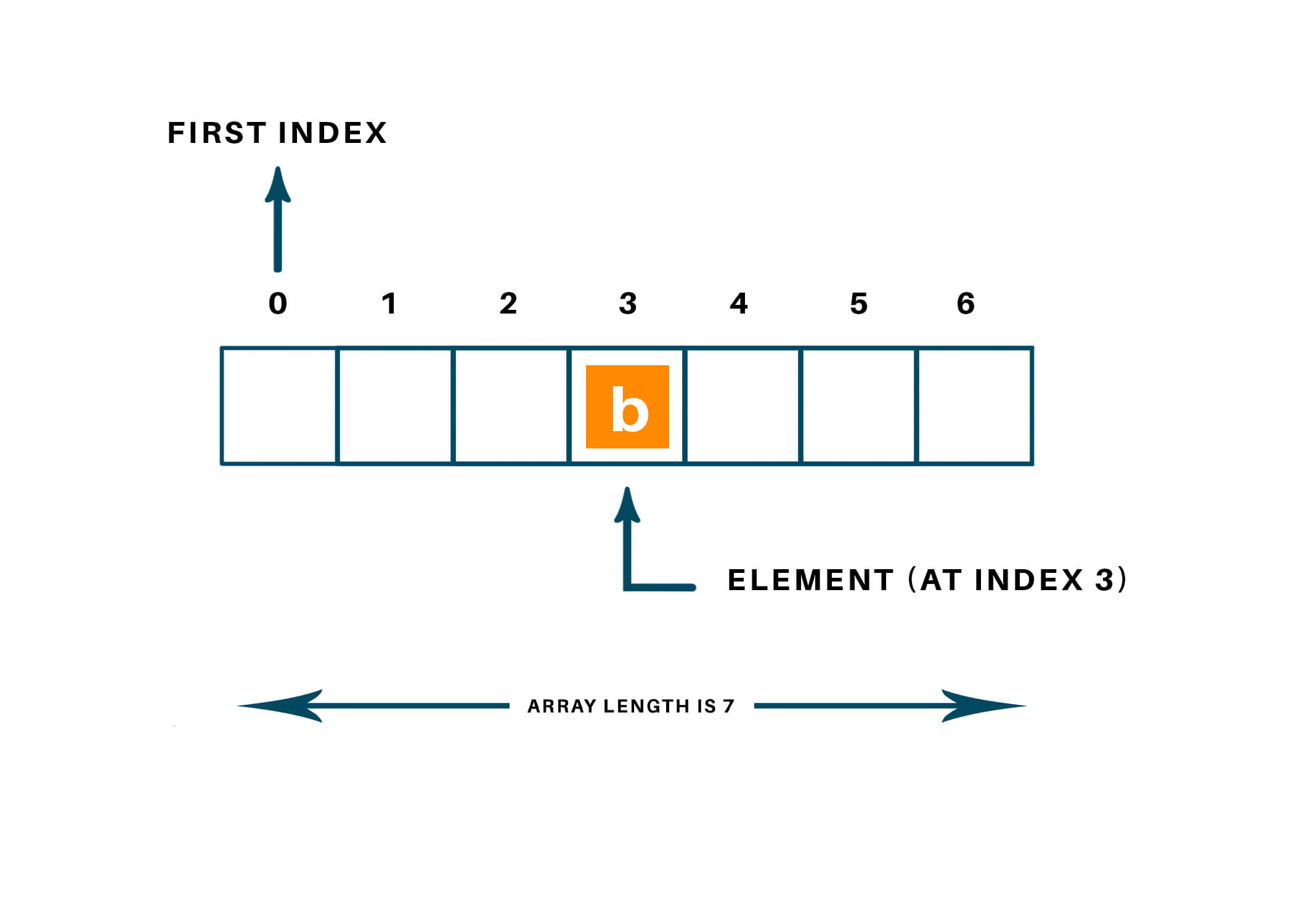# How to Initialize an Array in Python? (with Code)

In this article, we will study what is an array in python programming and how to initialize an array in python? We will understand different methods to do it. Also, we will see an example of each method along with its syntax to be followed and the output of the example given. So let's start!

## What is an Array in Python?

An array is a data structure in python programming that holds fix number of elements and these elements should be of the same data type. The main idea behind using an array of storing multiple elements of the same type. Most of the data structure makes use of an array to implement their algorithm. There is two important part of the array:

• Element: Each item store in the array is called an element.
• Index: Every element in the array has its own numerical value to identify the element.These elements allocate contiguous memory locations that allow easy modifications in data. In the python language, before using an array we need to declare a module named “array” using the keyword “import”.

## 3 Ways to Initialize an Array in Python

To use an array in the python language, there is a total of 3 ways to initialize it. We will look at all 3 ways on how to initialize an array in python. Let us study one by one below:

### Using for loop and Python range() Function

To initialize an array with the default value, we can use for loop and range() function in python language.

Syntax: [value for element in range(num)]

Python range() function takes a number as an argument and returns a sequence of number starts from 0 and ends by a specific number, incremented by 1 every time.

Python language for loop would place value 0(default value) for every item inside the array between range specified in the range() function.

Example:

```array=[]
array = [0 for i in range(3)]
print(array)
```

The output of the above code will be as shown below:

[0, 0, 0]

### Initializing array using python NumPy Module

Python language has many inbuilt libraries and functions which makes our task easier and simpler in comparison to other programming languages. NumPy module is one of them. NumPy module can be used to initialize the array and manipulate the data stored in it. The number.empty() function of the NumPy module creates an array of a specified size with the default value=” None”.

Syntax: numpy.empty(size,dtype=object)

Example:

```import numpy as np
array = np.empty(5, dtype=object)
print(array)
```

The output of the above code will be as shown below:

[None None None None None]

### Direct Methods to initialize an array

In the python language, we can directly initialize the elements inside an array using the below method.

Syntax: array-name = [default-value]*size

Example:

```arr_number =  * 3
print(arr_number)

arr_string = ['D'] * 3
print(arr_string)
```

The output of the above code is as shown below:

[1, 1, 1]

['D', 'D', 'D']

## Conclusion

Therefore, arrays are used to store the elements of the same data type and above are a few of the methods used to create or initialize an array in python programming. Further, we can perform many mathematical operations and modifications like adding, deleting, or updating an element inside the array with proper syntax to be followed. I hope you finally learned how to initialize an array in python.

### FavTutor - 24x7 Live Coding Help from Expert Tutors!